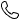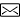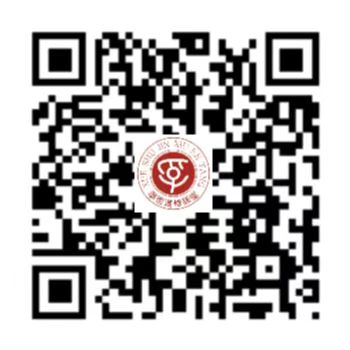0373-59399252851259250@qq.com# 基于四象限液晶器件的差分相衬成像系统

2 成像模型与理论

2.1　成像系统的构成

Fig.1  Structure diagram of liquid crystal differential phase contrast imaging system

2.2　定量差分相衬成像算法原理

o(r)=eiϕ(r)

， （1）

o(r)≈1+iϕ(r)

. （2）

I(uj)=S(uj)δ(u)|P(uj)|2+iS(uj)ϕ(u)×[P*(uj)P(u+uj)−P(uj)P*(u−uj)] .

（3）

I(u)=Bδ(u)+iΦ(u)PTF(u)

， （4）

B=∬S(uj)|P(uj)|2d2uj

. （5）

PTF(u)=∬S(uj)[P*(uj)P(u+uj)−P(uj)P*(u−uj)]d2uj

. （6）

IDPClr=Il−IrIl+Ir

. （7）

Il和Ir代表左半圆和右半圆照明时采集到的图像，IDPClr为样品在上下方向上的相位对比图，此时相位传递函数为：

F(Il−Ir)=iΦ(u)[PTFl(u)−PTFr(u)]

， （8）

F(Il+Ir)=(Bl+Br)δ(u)

. （9）

IDPClr(u)=iΦ(u)PTFlr(u)Bl+Br

. （10）

PTFDPClr(u)=∬Slr(uj)[P*(uj)P(u+uj)−P(uj)P*(u−uj)]d2uj∬Slr(uj)|P(uj)|2d2uj

. （11）

ϕ(r)=F−1⎧⎩⎨⎪⎪⎪⎪⎪⎪∑k[PTFDPC*k(u)⋅   IDPCk(u)]∑k∣∣PTFDPCk(u)∣∣2+α⎫⎭⎬⎪⎪⎪⎪⎪⎪

. （12）

2.3　液晶器件的设计

Fig.2  Liquid crystal devices used in this study

T=1−sin2[θ1+u2−−−−−√]1+u2

， （13）

u=4m2−1−−−−−−−√

（14）

Fig.3  Relationship between transmissivity and modulation of liquid crystal device

τon=η⋅   d2ΔεV2−π2k

（15）

τoff=η⋅   d2π2k

（16）

Fig.4  Relative transmittance versus time curve measured experimentally

3 实验结果与分析

3.1　微凸透镜阵列成像实验

Fig.5  Calculated phase diagram of microlens array

Fig.6  Comparison between theoretical curve and experimental curve

Δϕ=2πd(nm−nd)λ

. （17）

Pearson相关系数计算方式如式（18）所示：

ρ(A,B)=1N−1∑i=1N(Ai−μAσA)(Bi−μBσB)

. （18）

3.2　胚胎干细胞成像实验

Fig.7  Embryonic stem cell bright field image and reconstruction phase image

4 结论

#### 栏目分类

【免责声明】：中州期刊联盟所提供的信息资源如有侵权、违规，请及时告知。